Ex 4.3

Chapter 4 Class 9 Linear Equations in Two Variables
Serial order wise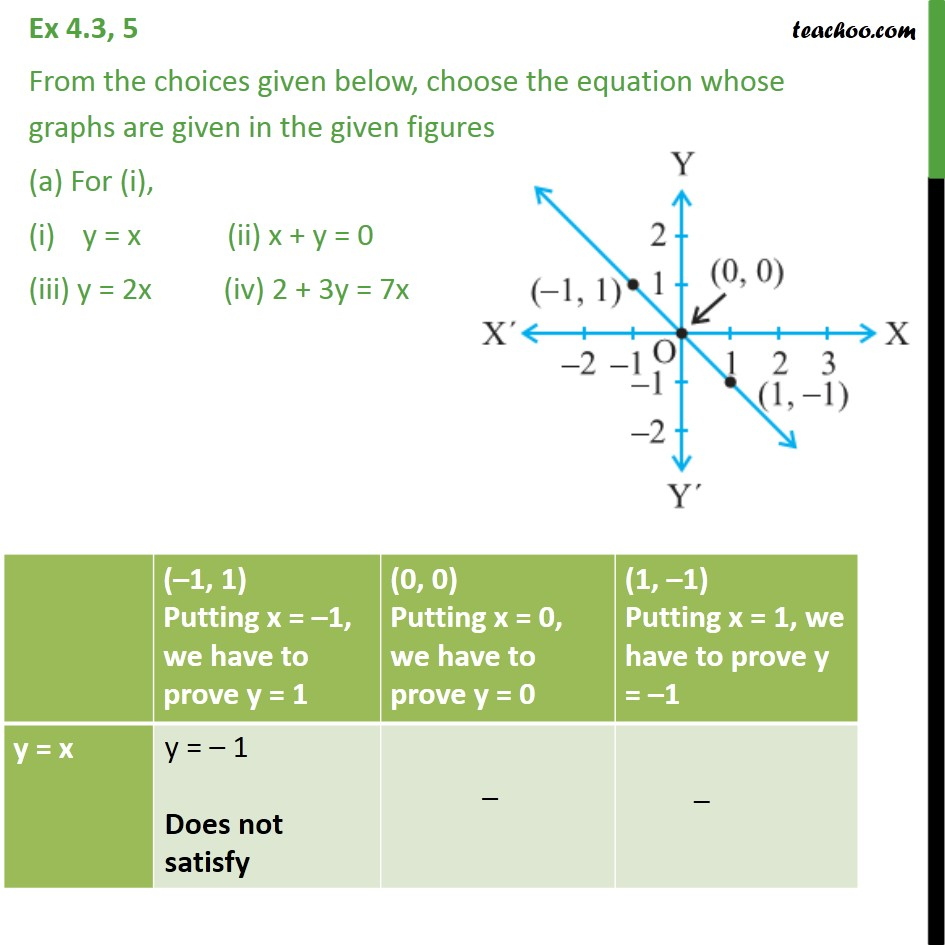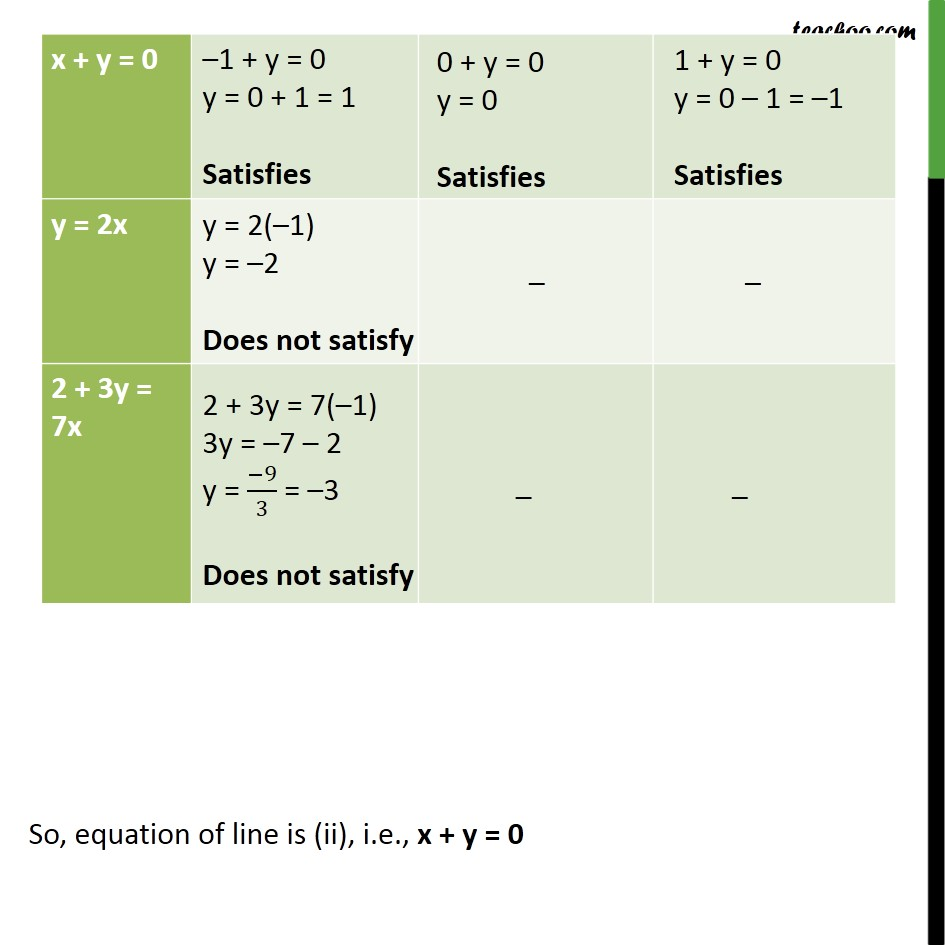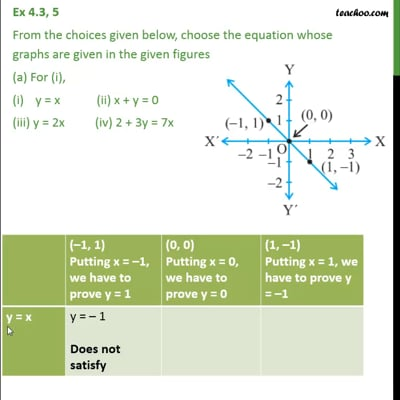This video is only available for Teachoo black users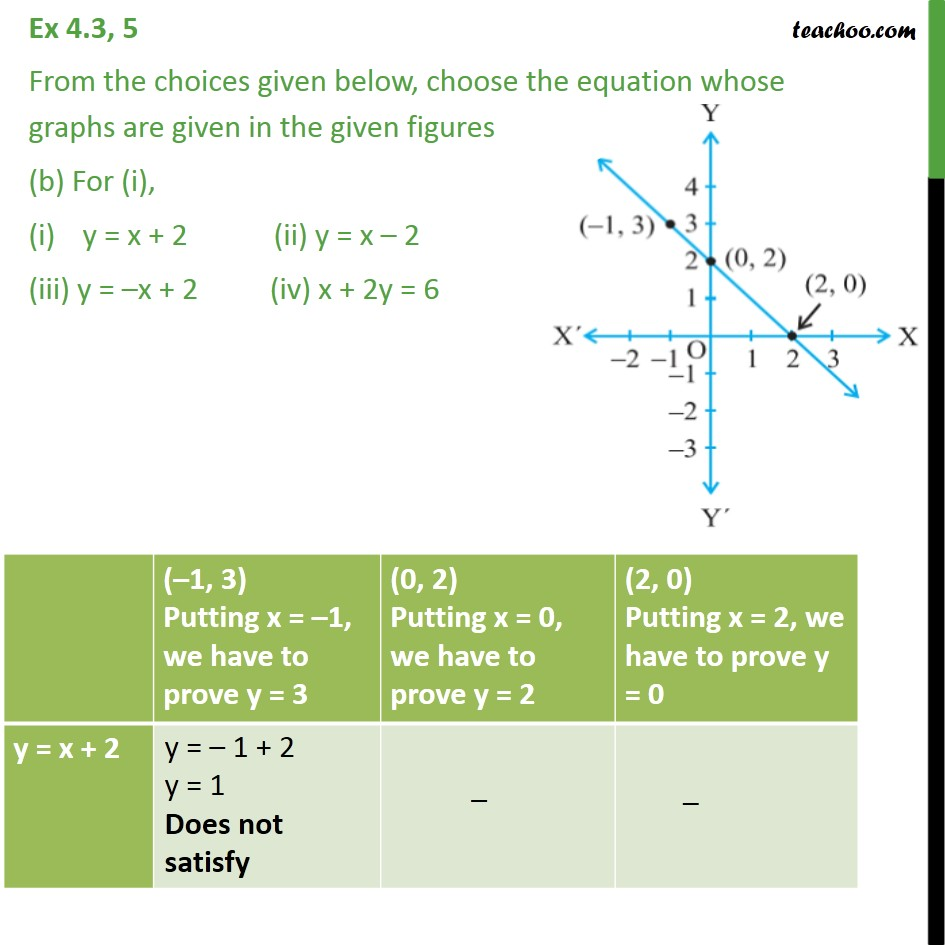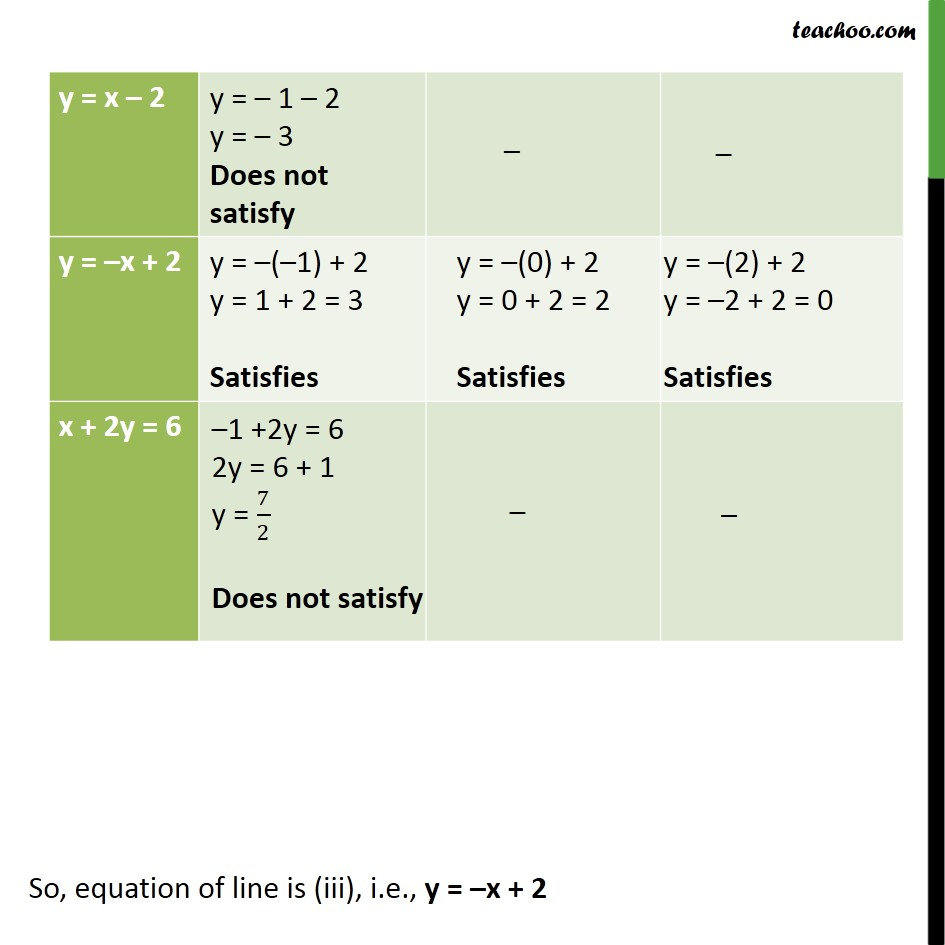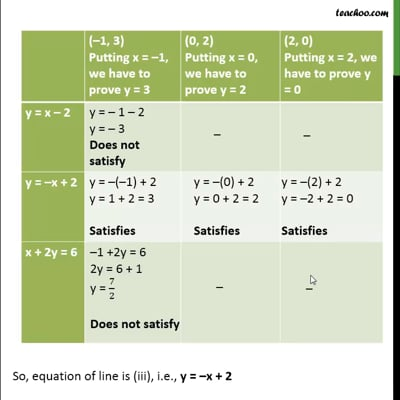This video is only available for Teachoo black users

Maths Crash Course - Live lectures + all videos + Real time Doubt solving!

### Transcript

Ex 4.3, 5 From the choices given below, choose the equation whose graphs are given in the given figures (a) For (i), y = x (ii) x + y = 0 (iii) y = 2x (iv) 2 + 3y = 7x So, equation of line is (ii), i.e., x + y = 0 Ex 4.3, 5 From the choices given below, choose the equation whose graphs are given in the given figures (b) For (i), y = x + 2 (ii) y = x 2 (iii) y = x + 2 (iv) x + 2y = 6 So, equation of line is (iii), i.e., y = x + 2